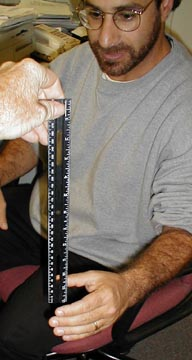Reaction Time

Think fastCatch the falling ruler starting with your fingers near 0 cm.

Introduction

You can find your reaction time by measuring the distance an object falls before you catch it.

Material

• a ruler
• two people

To Do and Notice

One person holds a ruler by its top, near the end with the largest number.
The other person places the centers of his or her fingers next to the 0 mark on the ruler ready to grab it. The second person should rest his or her hand on the edge of a table to prevent them from moving the hand up or down while the ruler falls.

The first person drops the ruler and the second person catches it.

Read the distance at the center of the fingers.

What's Going On?

Objects in free fall, like a ruler, accelerate toward the center of the earth with the acceleration g = 9.8 m/s2 or 32 ft/s2.

The equation for the time, t, it takes to free fall at the acceleration of gravity, g, over a distance,d, from rest is:

d = 1/2gt2

t = (2d/g)0.5

so in t = 0.1 second the ruler falls d = 4.9 * t2 = 4.9 * 0.12 = 0.049m = 4.9 cm
or d = 16 * t2 = 16 * 0.12 = 0.16 ft = 1.9 inches.

 t s d cm d in. 0.05 1.2 0.5 0.1 4.9 1.9 0.15 10.8 4.5 0.2 19.6 7.6 0.25 30.6 12.5

Or going from distance to time

t = (2/g)0.5 d0.5 = 0.45 * d0.5 = 0.45 * 0.010.5 = 0.045 s

 d cm t s 1 0.045 4 0.090 9 0.135 16 0.180 25 0.225

t = (2/g)0.5 d0.5 = (2/(32*12)) * d0.5 = 0.072 * 10.5 = 0.072 s

where I converted g from 32 ft/s2 to 384 in/s2

 d in t s 1 0.072 4 0.14 9 0.22 16 0.29 25 0.36

So What?

Average human reaction time from eyes to fingers is 0.16 s.

A car traveling 60 mi/hr is also going 96 km/hr or 88 ft/s or 27 m/s.

v = d/t speed v is distance divided by time.

Assume the car is traveling at a constant speed.
How far will it travel during one reaction time?
This will be the distance you travel before you even begin to step on the brakes.

d = v* t = 27 m/s * 0.16 s = 4.32 m

or d = 88 ft/s * 0.16 = 14 ft.

Going Further

Try to catch the falling ruler with your feet.

Notice the reaction time is longer.

How far will the car travel before you even begin to lift your foot off the accelerator?

Try to catch a dollar bill starting with your hands over the center of the bill. It is nearly impossible. Don't forget to brace your hand on the edge of a table so you don't cheat, and if you grab the bill before it is dropped, you lose.